# CRYPT-8 密钥分配与管理

## 目录

8.1 单钥加密体制的密钥分配 8.2 公钥加密体制的密钥管理 8.3 密钥托管 8.4 秘密分割

### 8.1 单钥加密体制的密钥分配

#### 8.1.2 秘钥分配案例

① A向KDC发出会话密钥请求。表示请求的消息由两个数据项组成，第1项是A和B的身份，第2项是这次业务的惟一识别符$N_1$，称$N_1$为一次性随机数，可以是时戳、计数器或随机数。每次请求所用的N1都应不同，且为防止假冒，应使敌手对N1难以猜测。因此用随机数作为这个识别符最为合适。

② KDC为A的请求发出应答。应答是由KA加密的消息，因此只有A才能成功地对这一消息解密，并且A可相信这一消息的确是由KDC 发出的。消息中包括A希望得到的两项内容：

• 一次性会话密钥$K_S$；
• A在①中发出的请求，包括一次性随机数$N_1$，目的是使A将收到的应答与发出的请求相比较，看是否匹配。

• 一次性会话密钥$K_S$；
• A的身份（例如A的网络地址）$ID_A$。

③ A存储会话密钥，并向B转发$E{K_B}[K_S||ID_A]$。因为转发的是由$K_B$加密后的密文，所以转发过程不会被窃听。B收到后，可得会话密钥$K_S$，并从$ID_A$可知另一方是A，而且还从$E{K_B}$知道$K_S$的确来自KDC。

#### 8.1.4 会话密钥的有效期

• 面向连接的协议，在连接未建立前或断开时，会话密钥的有效期可以很长。而每次建立连接时，都应使用新的会话密钥。如果逻辑连接的时间很长，则应定期更换会话密钥。
• 无连接协议（如面向业务的协议），无法明确地决定更换密钥的频率。为安全起见，用户每进行一次交换，都用新的会话密钥。然而这又失去了无连接协议主要的优势，即对每个业务都有最少的费用和最短的延迟。比较好的方案是在某一固定周期内或对一定数目的业务使用同一会话密钥。

#### 8.1.5 无中心的密钥控制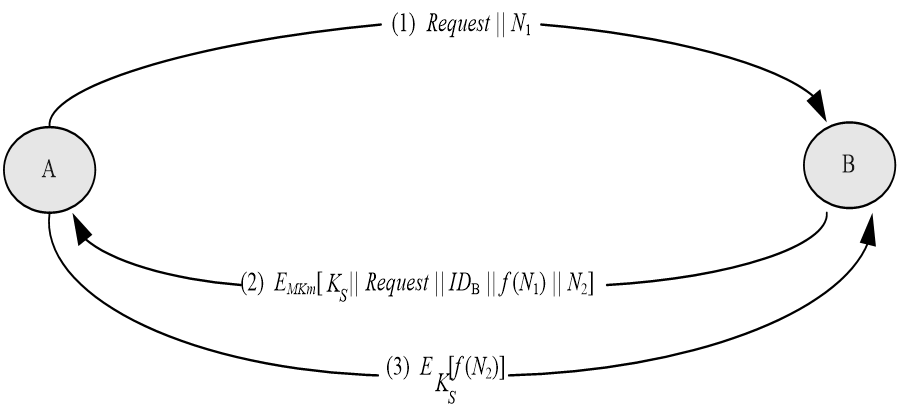无中心的密钥分配

#### 8.1.6 密钥的控制使用

• 密钥可根据其不同用途分为会话密钥和主密钥两种类型，会话密钥又称为数据加密密钥，主密钥又称为密钥加密密钥。由于密钥的用途不同，因此对密钥的使用方式也希望加以某种控制。
• 如果主密钥泄露了，则相应的会话密钥也将泄露，因此主密钥的安全性应高于会话密钥的安全性。一般在密钥分配中心以及终端系统中主密钥都是物理上安全的，如果把主密钥当作会话密钥注入加密设备，那么其安全性则降低。

• 一个比特表示这个密钥是会话密钥还是主密钥；
• 一个比特表示这个密钥是否能用于加密；
• 一个比特表示这个密钥是否能用于解密；
• 其他比特无特定含义，留待以后使用。

• 第一，标签的长度被限制为8比特，限制了它的灵活性和功能；
• 第二，由于标签是以密文形式传送，只有解密后才能使用，因而限制了对密钥使用的控制方式。

(2) 控制矢量 这一方案比上一方案灵活。方案中对每一会话密钥都指定了一个相应的控制矢量，控制矢量分为若干字段，分别用于说明在不同情况下密钥是被允许使用还是不被允许使用，且控制矢量的长度可变。控制矢量是在KDC产生密钥时加在密钥之中的，过程由图5.3(a)所示。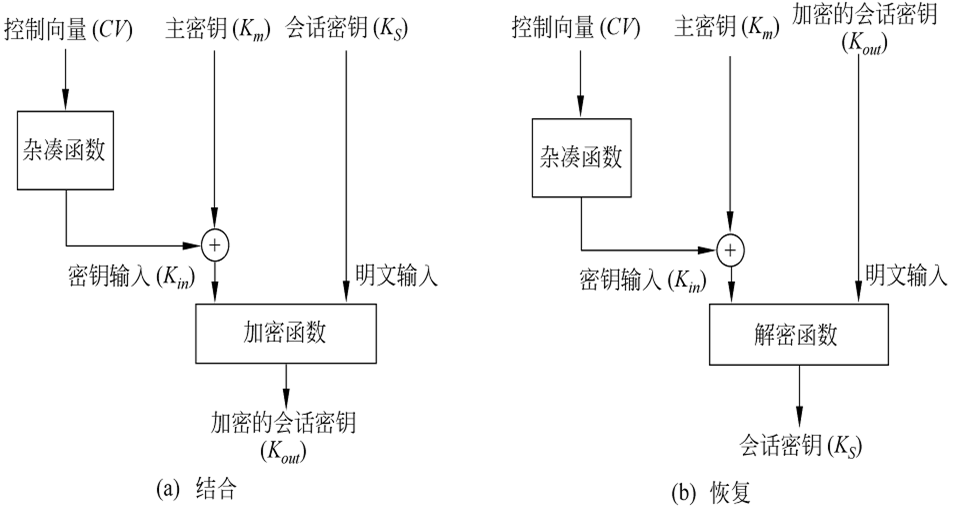KDC在向用户发送会话密钥时，同时以明文形式发送控制矢量。用户只有使用与KDC共享的主密钥以及KDC发送来的控制矢量才能恢复会话密钥，因此还必须保留会话密钥和它的控制矢量之间的对应关系。 与使用8比特的密钥标签相比，使用控制矢量有两个优点:

• 第一，控制矢量的长度没有限制，因此可对密钥的使用施加任意复杂的控制；
• 第二，控制矢量始终是以明文形式存在，因此可在任一阶段对密钥的使用施加控制。

### 8.2 公钥加密体制的密钥管理

• 一是公钥密码体制所用的公开密钥的分配，
• 二是如何用公钥体制来分配单钥密码体制所需的密钥。

#### 8.2.1 公钥的分配

1. 公开发布

2. 公用目录表

3. 公钥管理机构

① 用户A向公钥管理机构发送一个带时戳的消息，消息中有获取用户B的当前公钥的请求。 ② 管理机构对A的请求作出应答，应答由一个消息表示，该消息由管理机构用自己的秘密钥$SK_{AU}$加密，因此A能用管理机构的公开钥解密，并使A相信这个消息的确是来源于管理机构。

• B的公钥$PK_B$，A可用之对将发往B的消息加密；
• A的请求，用于A验证收到的应答的确是对相应请求的应答，且还能验证自己最初发出的请求在被管理机构收到以前是否被篡改；
• 最初的时戳，以使A相信管理机构发来的消息不是一个旧消息，因此消息中的公开钥的确是B当前的公钥。

③ A用B的公开钥对一个消息加密后发往B，这个消息有两个数据项: 一是A的身份IDA，二是一个一次性随机数$N_1$，用于惟一地标识这次业务。 ④ B以相同方式从管理机构获取A的公开钥（与步骤①、②类似）。这时，A和B都已安全地得到了对方的公钥，所以可进行保密通信。然而，他们也许还希望有以下两步，以认证对方。

⑤ B用$PK_A$对一个消息加密后发往A，该消息的数据项有A的一次性随机数$N_1$和B产生的一个一次性随机数$N_2$。因为只有B能解密③的消息，所以A收到的消息中的N1可使其相信通信的另一方的确是B。 ⑥ A用B的公开钥对$N_2$加密后返回给B，可使B相信通信的另一方的确是A。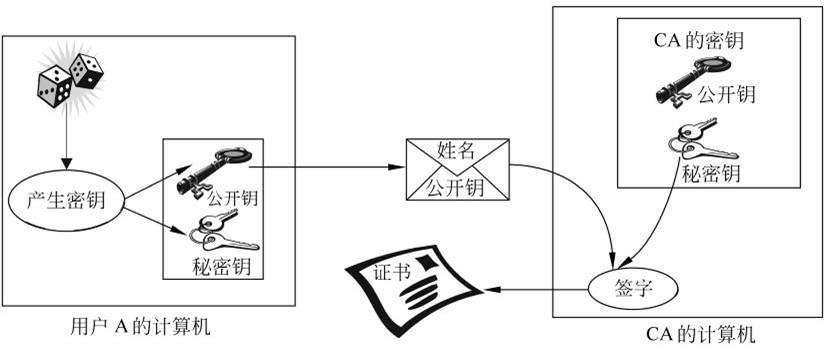证书的产生过程

#### 8.2.2 用公钥加密分配单钥密码体制的密钥

1. 简单分配 图5.6表示简单使用公钥加密算法建立会话密钥的过程，如果A希望与B通信，可通过以下几步建立会话密钥： ① A产生自己的一对密钥{PKA,SKA}，并向B发送PKA||IDA，其中IDA表示A的身份。 ② B产生会话密钥KS，并用A的公开钥PKA对KS加密后发往A。 ③ A由DSKA[EPKA[KS]]恢复会话密钥。因为只有A能解读KS，所以仅A、B知道这一共享密钥。 ④ A销毁{PKA,SKA}，B销毁PKA。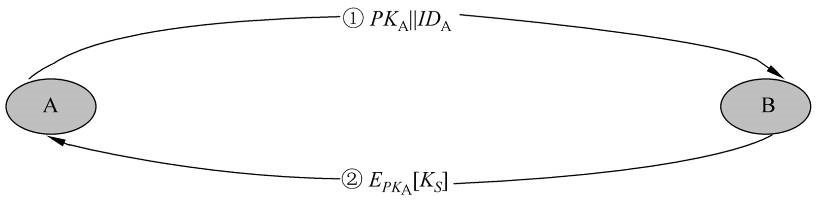简单使用公钥加密算法建立会话密钥

A、B现在可以用单钥加密算法以$K_S$作为会话密钥进行保密通信，通信完成后，又都将$K_S$销毁。这种分配法尽管简单，但却由于A、B双方在通信前和完成通信后，都未存储密钥，因此，密钥泄露的危险性为最小，且可防止双方的通信被敌手监听。

1. 具有保密性和认证性的密钥分配 图中所示的密钥分配过程具有保密性和认证性，因此既可防止被动攻击，又可防止主动攻击。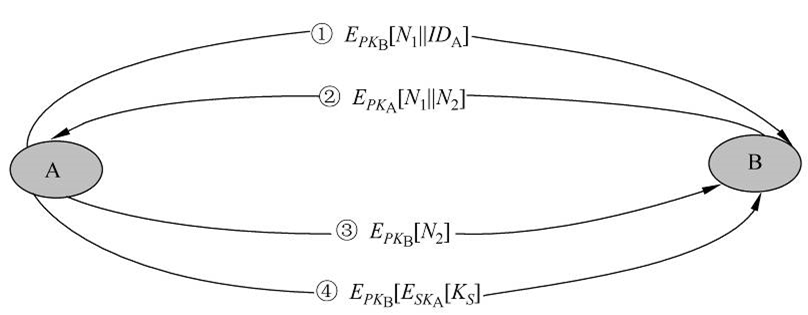③ A用B的公钥$PKB$对$N_2$加密后返回给B，以使B相信对方的确是A。 ④ A选一会话密钥$K_S$，然后将$M=E{PKB}[E{SKA}[K_S]]$发给B，其中用B的公开钥加密是为保证只有B能解读加密结果，用A的秘密钥加密是保证该加密结果只有A能发送。 ⑤ B以$D{PKA}[D{SK_B}[M]]$恢复会话密钥。

#### 8.2.3 Diffie-Hellman密钥交换

Diffie-Hellman密钥交换是W. Diffie和M. Hellman于1976年提出的第一个公钥密码算法，已在很多商业产品中得以应用。算法的惟一目的是使得两个用户能够安全地交换密钥，得到一个共享的会话密钥，算法本身不能用于加、解密。 算法的安全性基于求离散对数的困难性。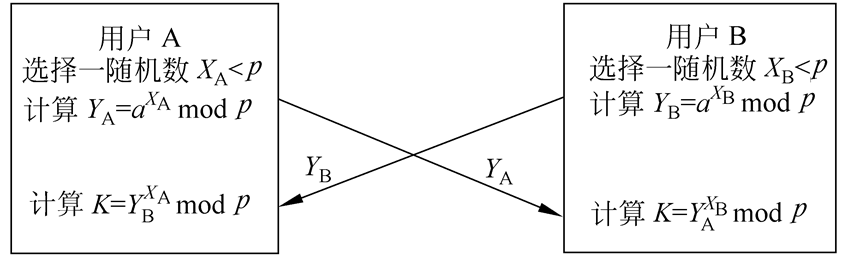Diffie-Hellman的密钥交换过程

\begin{align} (Y_B)^{X_A}\space mod\space p &=(a^{X_B}\space mod\space p)^{X_A}\space mod\space p=(a^{X_B} )^{X_A}\space mod\space p \ & = a^{X_BX_A}\space mod\space p = (a^{X_A})^{X_B}\space mod\space p \ & =(a^{X_A}\space mod\space p)^{X_B}\space mod = (Y_A)^{X_B}\space mod\space p \ \end{align}$K= (Y_B)^{X_A\space} mod\space 97=4436\space mod\space 97=75$

$K= (Y_A)^{X_B}\space mod\space 97=5058\space mod\space 97=75$

### 8.3 密钥托管

#### 8.3.1 美国托管加密标准简介

1993年4月，美国政府为了满足其电信安全、公众安全和国家安全，提出了托管加密标准EES(escrowed encryption standard)，该标准所使用的托管加密技术不仅提供了强加密功能，同时也为政府机构提供了实施法律授权下的监听功能。这一技术是通过一个防窜扰的芯片(称为Clipper芯片)来实现的。

1. Skipjack算法 Skipjack算法是一个单钥分组加密算法，密钥长80比特，输入和输出的分组长均为64比特。可使用4种工作模式：电码本模式，密码分组链接模式，64比特输出反馈模式，1、8、16、32或64比特密码反馈模式。

2. 托管加密芯片

Skipjack算法以及在法律授权下对加密结果的存取是通过防窜扰的托管加密芯片来实现的。芯片装有以下部分：

• Skipjack算法；
• 80比特的族密钥KF(family key)，同一批芯片的族密钥都相同;
• 芯片单元识别符UID(unique identifier);
• 80比特的芯片单元密钥KU(unique key),它是两个80比特的芯片单元密钥分量(KU1,KU2)的异或;
• 控制软件。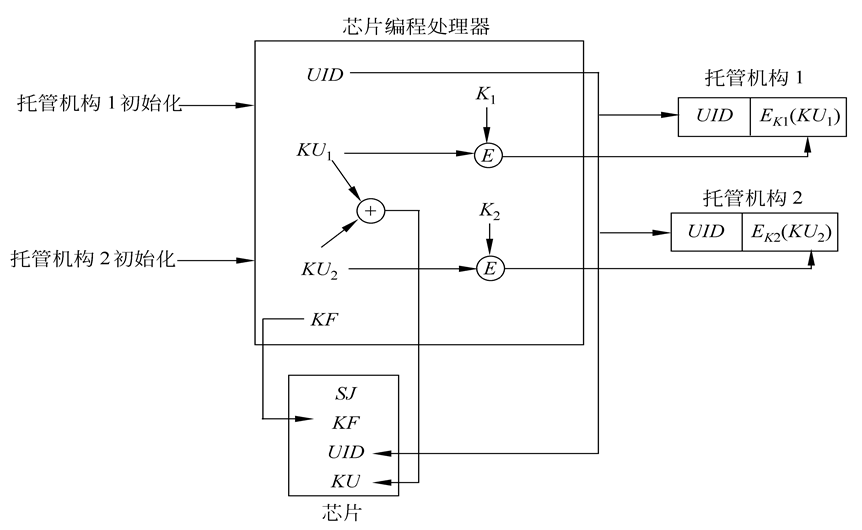3.用托管加密芯片加密

80比特的会话密钥KS建立后，被传送给加密芯片，用于与初始化向量IV（由芯片产生）一起产生LEAF。控制软件使用芯片单元密钥KU加密KS，然后将加密后的结果和芯片识别符UID、认证符A链接，再使用公共的族密钥KF加密以上链接的结果而产生LEAF。4. 法律实施存取 政府机构在进行犯罪调查时，为了监听被调查者的通信，首先必须取得法院的许可证书，并将许可证书出示给通信服务的提供者（电信部门），并从电信部门租用线路用来截取被监听者的通信。如果被监听者的通信是经过加密的，则被截获的通信首先通过一个政府控制的解密设备，如上一张图所示，其中D表示解密。解密设备可识别由托管芯片加密的通信，取出LEAF和IV，并使用族密钥KF解密LEAF以取出芯片识别符UID和加密的会话密钥$E_{KU}(KS)$。

#### 8.3.2 密钥托管密码体制的组成成分

EES提出以后，密钥托管密码体制受到了普遍关注，已提出了各种类型的密钥托管密码体制，包括软件实现的、硬件实现的、有多个委托人的、防用户欺诈的、防委托人欺诈的等。密钥托管密码体制从逻辑上可分为3个主要部分：用户安全成分USC(user security component)、密钥托管成分KEC(key escrow component)和数据恢复成分DRC(data recovery component)。三者的关系如图5.12所示，USC用密钥KS加密明文数据，并且在传送密文时，一起传送一个数据恢复域DRF（data recovery field）。DRC使用包含在DRF中的信息及由KEC提供的信息恢复明文。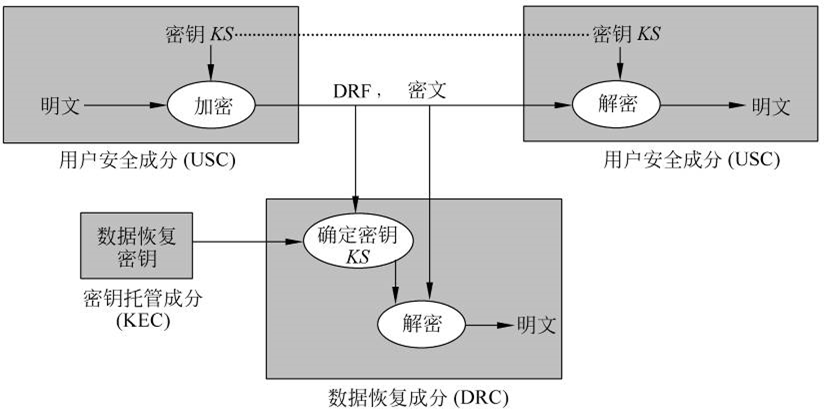### 8.4 秘密分割

#### 8.4.2 Shamir门限方案

Shamir门限方案是基于多项式的Lagrange插值公式的。插值是古典数值分析中的一个基本问题，问题如下：已知一个函数$\varphi(x)$在k个互不相同的点的函数值$\varphi(x_i)(i=1,2,...,k)$，寻求一个满足$f(x_i)=\varphi (x_i)(i=1,2,...,k)$的函数$f(x)$，用来逼近$\varphi(x)$。$f(x)$称为$\varphi (x)$的插值函数，$f(x)$可取自不同的函数类，既可为代数多项式，也可为三角多项式或有理分式。若取$f(x)$为代数多项式，则称差值问题为代数插值，$f(x)$称为$\varphi (x)$的插值多项式。常用的代数插值有Lagrange插值、Newton插值、Hermite插值。

Lagrange插值 已知$\varphi(x)$在k个互不相同的点的函数值$\varphi(x_i)(i=1,2,...,k)$，可构造k-1次插值多项式为

$$f(x)=\sum{j=1}^{k}\varphi(x_j)\prod{i=1,i\neq j}^{k}\frac{x-x_i}{x_j-x_i}$$

$f(x)=a0+a_1x+...+a{k-1}x^{k-1}$

n个参与者记为$P_1,P_2,...,P_n,P_i$分配到的子密钥为$f(i)$。如果任意$k$个参与者

$$P{i_1},...,P{i_k},(1\leq i_1\leq i_2\leq ...\leq i_k\leq n)$$ 要想得到秘密s，可使用${(i_l,f(i_l))|l=1,…,k}$构造如下的线性方程组：

$$\left{ \begin{array}{c} a0+a_1(i_1)+...+a{k-1}(i1)^{k-1}=f(i_1) \ a_0+a_1(i_2)+...+a{k-1}(i2)^{k-1}=f(i_2) \ ...... \ a_0+a_1(i_k)+...+a{k-1}(i_k)^{k-1}=f(i_k) \ \end{array} \right.$$

$$f(x)=\sum^{k}{j=i}f(i_j)\prod^{k}{l=1,l\neq j}\frac{x-i_l}{i_j-i_l}\space mod\space q$$

$$s=(-1)^{k-1}\sum^{k}{j=1}f(i_j)\prod^{k}{l=1,l\neq j}\frac{i_l}{i_j-i_l}\space mod\space q$$

$f(x)=(7x^2+2x+11)\space mod\space 19$

$f(1)=(7+2+11)\space mod\space 19=20\space mod\space 19=1$ $f(2)=(28+4+11)\space mod\space 19=43\space mod\space 19=5$ $f(3)=(63+6+11)\space mod\space 19=80\space mod\space 19=4$ $f(4)=(112+8+11)\space mod\space 19=131\space mod\space 19=17$ $f(5)=(175+10+11)\space mod\space 19=196\space mod\space 19=6$

\begin{align} 5\times \frac{(x-3)(x-5)}{(2-3)(2-5)}&=5\times\frac{(x-3)(x-5)}{3}=5\times(3^{-1}\space mod\space 19)\times(x-3)(x-5) \ & = 5\times 13\times (x-3)(x-5)=65(x-3)(x-5) \end{align}

\begin{align} 4\times \frac{(x-2)(x-5)}{(3-2)(3-5)}&=4\times\frac{(x-2)(x-5)}{-2}=4\times((-2)^{-1}\space mod\space 19)\times(x-2)(x-5) \ & = 4\times 9\times (x-2)(x-5)=36(x-2)(x-5) \end{align}

\begin{align} 6\times \frac{(x-2)(x-3)}{(5-2)(5-3)}&=6\times\frac{(x-2)(x-3)}{6}=6\times(6^{-1}\space mod\space 19)\times(x-2)(x-3) \ & = 6\times 16\times (x-2)(x-3)=96(x-2)(x-3) \end{align}

\begin{align} f(x)&=[65(x-3)(x-5)+36(x-2)(x-2)(x-5)+96(x-2)(x-3)]\space mod\space 19 \ & =[8(x-3)(x-5)+17(x-2)(x-5)+(x-2)(x-3)]\space mod\space 19 \ & =(26x^2-188x+296)\space mod\space 19 \ & =7x^2+2x+11 \end{align}

#### 8.4.3 基于中国剩余定理的门限方案

$\left{ \begin{array}{l} M{i_j}=\frac{M}{m{ij}} \ N{ij}\equiv M{ij}^{-1}\space mod\space m{ij} \ y{ij}=s{ij}M{ij}N{i_j} \ \end{array} \right.$